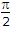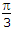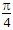# Electronics and Communication Engineering - Power Electronics

### Exercise :: Power Electronics - Section 7

26.

An SCR is triggered at 80° in the positive half cycle, only. The rms anode current is 25 A. If the firing angle is changed to 160°, the rms current is likely to be

 A. 25 A B. 12.5 A C. less than 25 A D. less than 12.5 A

Explanation:

No answer description available for this question. Let us discuss.

27.

The output wave of a cycloconverter consists of a number of segments derived from the input wave.

 A. True B. False

Explanation:

No answer description available for this question. Let us discuss.

28.

A class A chopper has an input voltage of 200 V. Ton = 1.5 ms and Toff = 4.5 ms. The duty cycle, average output voltage and ripple factor respectively are

 A. 0.5, 100 V and 1 B. 0.25, 50 V and 3 C. 0.25, 50 V and 1 D. 0.5, 100 V and 3

Explanation:

No answer description available for this question. Let us discuss.

29.

In a parallel inverter the turn off time tq of thyristor and values of commutating elements L and C are related as

 A. tq = pLC B. tq =LC C. tq =LC D. tq =LC

Explanation:

No answer description available for this question. Let us discuss.

30.

A TRIAC can be turned on :

 A. by applying a positive signal to gate B. by applying a negative signal to gate C. by applying either positive or negative signal to gate D. none of the above